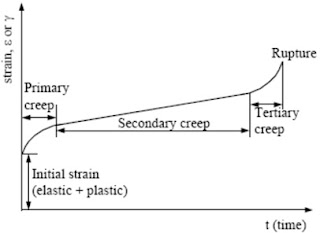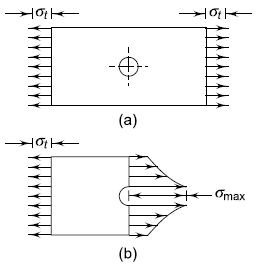### Design of Machine Elements - short answer type questions from AMIE exams (Summer 2019)

Explain the following terms:

### Stress concentration factor.

In order to consider the effect of stress concentration and find out localized stresses, a factor called the stress concentration factor is used. It is denoted by Kt and defined as,

orwhere σ0 and τ0 are stresses determined by elementary equations and σmax and τmax are localized stresses at the discontinuities. The subscript t denotes the ‘theoretical’ stress concentration factor.

### Notch sensitivity factor

Notch sensitivity is defined as the susceptibility of a material to succumb to the damaging effects of
stress raising notches in fatigue loading. The notch sensitivity factor q is defined as

### Fatigue strength reduction factor

This factor accounts for geometrical stress concentration factors due to thickness variations; weld geometries, surface notches or environmental conditions. The range of factor Kg is between 1 and 4, with its minimum value for smooth geometrical shapes and its maximum for 90 degree welded corners and fillet welds.

### State and explain the efficiency of the screw.

The efficiency of the power screw is given bySee the following figure of square thread screw

### Different types of rolling contact bearing.

Following are the two types of rolling contact bearings:
• Ball bearings
• Roller bearings.

The ball and roller bearings consist of an inner race which is mounted on the shaft or journal
and an outer race which is carried by the housing or casing.
The rolling contact bearings, depending upon the load to be carried, are classified as
• Thrust bearings.

### What are the design criteria of brakes?

Before starting designing any brake we need to calculate the following braking parameters
Step 1  Calculate the kinetic energy of your vehicle
KE=1/2mv2
Step 2  Stopping distance D
D = v2/(2μg)
μ = coefficient friction b/w road surface and tire.
Step 3  For deceleration (m/s2)
V2= u+ 2aS
U = initial velocity during braking
V = final velocity after braking
Here a will be - ve which is deceleration and S is the stopping distance.
Step 4  Calculate the time required (sec)
Use, v = u + at
Step 5  Braking force
Total Braking force = K. E./Stopping distance
Then to calculate the braking force on each wheel divide the total braking force by no. of wheels.
Step 7  Braking torque = braking force on each wheel(N) x  radius of the wheel.
It is also given by
E = Mtθ
where
E = total energy absorbed by the brake (J)
Mt = braking-torque (N-m)
θ = angle through which the brake drum rotates during the braking period (rad)

### Write the formula for the efficiency of the screw jack.

The formula for the efficiency of the screw jack isFor maximum efficiency,Maximum efficiency is### Define malleability

The malleability of a material is its ability to be flattened into thin sheets without cracking by hot or cold working. Aluminium, copper, tin, lead, steel, etc. are malleable metals.
Lead can be readily rolled and hammered into thin sheets but can not be drawn into wire. Ductility is a tensile property, whereas malleability is a compressive property. Malleability increases with an increase in temperature.

### Define creep.

Creep is the tendency of a solid material to move slowly or deform permanently under the influence of persistent mechanical stresses. It can occur as a result of long-term exposure to high levels of stress that are still below the yield strength of the material. Creep is more severe in materials that are subjected to heat for long periods and generally increases as they near their melting point.A creep curve

### What do you understand by stress concentration?

In practice, discontinuities and abrupt changes in cross-section are unavoidable due to certain features of the component such as oil holes and grooves, keyways and splines, screw threads and shoulders. Therefore, it cannot be assumed that the cross-section of the machine component is uniform. Under these circumstances, the ‘elementary’ equations do not give correct results.

Let us consider a  plate with a small circular hole.Stress concentration

When the plate is loaded at the edges, It is observed that there is a sudden rise in the magnitude of stresses in the vicinity of the hole. The localized stresses in the neighbourhood of the hole are far greater than the stresses obtained by elementary equations.

Stress concentration is defined as the localization of high stresses due to the irregularities present in the component and abrupt changes of the crosssection.

---
The study material for AMIE/B Tech/Junior Engineer exams is available at https://amiestudycircle.com